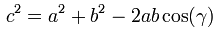### Compton Scattering Paper Mislabeled? It's Obvious... Not

This installment of “It’s Obvious. Not!” looks at:

Periodical: “Physical Review” Volume: 21 Page: 483, 486
Title: "A Quantum Theory of the Scattering of X-Rays by Light Elements"
Author: Arthur H. Compton

Excerpt from page 486:In the above excerpt, Compton discusses how to calculate the momentum of an electron that caused x-ray or gamma scattering. The momentum added to the electron is the momentum of the incident photon minus the momentum of the scattered photon.

Problem: The angle of scattering, (theta), appears to be mislabeled in the above figure vs. the usage of the angle in formula 1.

In formula 1, Compton calculates the magnitude of the electron momentum as the vector difference of the momentum of the incident and scattered photons. To subtract two vectors, you place their tails together, the resulting vector that points from the head of the second to the head of the first is the difference vector as shown below and described in this Wikipedia article.To get the magnitude of the difference vector given the magnitude of the other two vectors, you can use the Law of Cosines and the figure shown below.And that's just what Compton does to arrive at formula 1... almost. If you read the excerpt from Compton's article carefully, you'll see that the last term of the formula for the electron's momentum magnitude is added rather than subtracted. Per normal, this unexpected change makes the derivations further along in the paper come out quite nicely. However, if you perform the magnitude calculation with the labeled value of theta, the last term has to be subtracted, rather than added, per the law of cosines.

To make everything comes out correctly, it appears that the angle that must be used is not theta, but the supplementary angle, pi minus theta. The cosine of pi minus theta is the negative of the cosine of theta which cancels Compton's sign change in the last term. Compton may have indicated this choice of angles in the text with the phrase:Have any thoughts on this? Please let me know. I might be wrong, in which case it would be great to find out the real explanation!dark_faust said…
You're explanation seems the most likely, (-)(-) = +

IMHO: Choice of angle (or coordinates) was the culprit for most of these sign problems. This is were the professors would say, "It is a trivial exercise to show that a judicious choice of angles leads to the direct solution."

### Cool Math Tricks: Deriving the Divergence, (Del or Nabla) into New (Cylindrical) Coordinate Systems

The following is a pretty lengthy procedure, but converting the divergence, (nabla, del) operator between coordinate systems comes up pretty often. While there are tables for converting between common coordinate systems, there seem to be fewer explanations of the procedure for deriving the conversion, so here goes!

What do we actually want?

To convert the Cartesian nabla

to the nabla for another coordinate system, say… cylindrical coordinates.

What we’ll need:

1. The Cartesian Nabla:

2. A set of equations relating the Cartesian coordinates to cylindrical coordinates:

3. A set of equations relating the Cartesian basis vectors to the basis vectors of the new coordinate system:

How to do it:

Use the chain rule for differentiation to convert the derivatives with respect to the Cartesian variables to derivatives with respect to the cylindrical variables.

The chain rule can be used to convert a differential operator in terms of one variable into a series of differential operators in terms of othe…

### The Valentine's Day Magnetic Monopole

There's an assymetry to the form of the two Maxwell's equations shown in picture 1.  While the divergence of the electric field is proportional to the electric charge density at a given point, the divergence of the magnetic field is equal to zero.  This is typically explained in the following way.  While we know that electrons, the fundamental electric charge carriers exist, evidence seems to indicate that magnetic monopoles, the particles that would carry magnetic 'charge', either don't exist, or, the energies required to create them are so high that they are exceedingly rare.  That doesn't stop us from looking for them though!

Keeping with the theme of Fairbank and his academic progeny over the semester break, today's post is about the discovery of a magnetic monopole candidate event by one of the Fairbank's graduate students, Blas Cabrera.  Cabrera was utilizing a loop type of magnetic monopole detector.  Its operation is in concept very simpl…